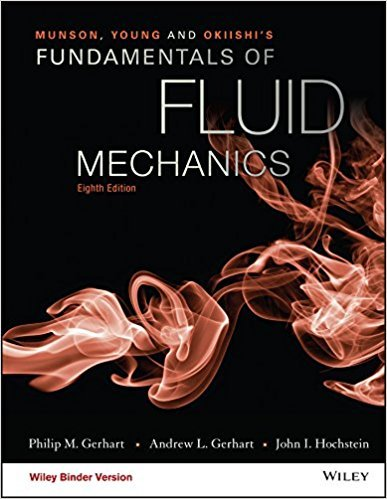×
Get Full Access to Fundamentals Of Fluid Mechanics - 8 Edition - Chapter 11.6 - Problem 11.41
Get Full Access to Fundamentals Of Fluid Mechanics - 8 Edition - Chapter 11.6 - Problem 11.41

×

# Air flows isentropically through a duct to a section wherep1 = 25 kPa, T1 = 300K, and V1ISBN: 9781119080701 456

## Solution for problem 11.41 Chapter 11.6

Fundamentals of Fluid Mechanics | 8th Edition

• Textbook Solutions
• 2901 Step-by-step solutions solved by professors and subject experts
• Get 24/7 help from StudySoup virtual teaching assistantsFundamentals of Fluid Mechanics | 8th Edition

4 5 1 430 Reviews
13
5
Problem 11.41

Air flows isentropically through a duct to a section wherep1 = 25 kPa, T1 = 300K, and V1 = 900 m/s. For these conditions:(a) Determine the stagnation conditions for the flow.(b) What is the Mach number at station 1? Show a Ts diagramdisplaying stagnation and static conditions.(c) Is the flow choked? Is the throat behind or ahead of section 1?Label this state on the Ts diagram.

Step-by-Step Solution:
Step 1 of 3

Chapter 18: The Heart – Cardiac Output (Continued)  Under normal conditions: pressure in ventricle is higher than blood in the aorta  In afterload (hypertension): pressure in aorta is higher than blood in aorta o Provides a hard time for the ventricle to push open valve flaps 1)  ESV;  SV =  Cardiac Output  Factors Influencing Heart Rate  Chronotrope: chemicals that have an effect on heart rate o Positive Chronotropic Agents =  SV o Negative Chronotropic Agents =  SV o Autonomic Nervous System 1) Sympathetic NS  Stress response, fight of flight = Positive Chronotopes  Activation of Sympathetic NS  NT (neurotransmitter): Norepinephrine binds to beta- 1 receptors on SA Node o Influences pacemaker potential o Makes the SA Node fire faster;  HR (heart rate) + 2+  Increases Na and causes Ca influx o Fiber innervating the myocardium in contractile cardiac muscle cells o  Contractility;  ESV =  SV  Important to Note: SV does NOT decline as it would if only  HR; contractility serve as a compensating factor!  Releases NT Epinephrine from the Adrenal Medulla i

Step 2 of 3

Step 3 of 3

##### ISBN: 9781119080701

The answer to “Air flows isentropically through a duct to a section wherep1 = 25 kPa, T1 = 300K, and V1 = 900 m/s. For these conditions:(a) Determine the stagnation conditions for the flow.(b) What is the Mach number at station 1? Show a Ts diagramdisplaying stagnation and static conditions.(c) Is the flow choked? Is the throat behind or ahead of section 1?Label this state on the Ts diagram.” is broken down into a number of easy to follow steps, and 66 words. This textbook survival guide was created for the textbook: Fundamentals of Fluid Mechanics, edition: 8. Fundamentals of Fluid Mechanics was written by and is associated to the ISBN: 9781119080701. This full solution covers the following key subjects: . This expansive textbook survival guide covers 112 chapters, and 1357 solutions. Since the solution to 11.41 from 11.6 chapter was answered, more than 257 students have viewed the full step-by-step answer. The full step-by-step solution to problem: 11.41 from chapter: 11.6 was answered by , our top Science solution expert on 03/16/18, 03:21PM.

## Discover and learn what students are asking

Chemistry: The Central Science : Nuclear Chemistry
Give the symbol for (a) a proton, (b) a beta particle, (c) a positron.

Unlock Textbook Solution